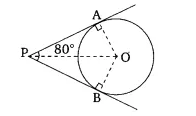# 3.   If tangents PA and PB from a point P to a circle with centre O are inclined to each other at angle of , then  POA is equal to      (A) 50°      (B) 60°      (C) 70°      (D) 80°

M manish

The correct option is (A)It is given that,  tangent PA and PB from point P inclined at
In triangle OAP and OBP

OA =OB (radii of the circle)
PA = PB (tangents of the circle)

Therefore, by  SAS congruence

By CPCT,
Now, OPA = 80/4 = 40

In  PAO,

= 50

Exams
Articles
Questions